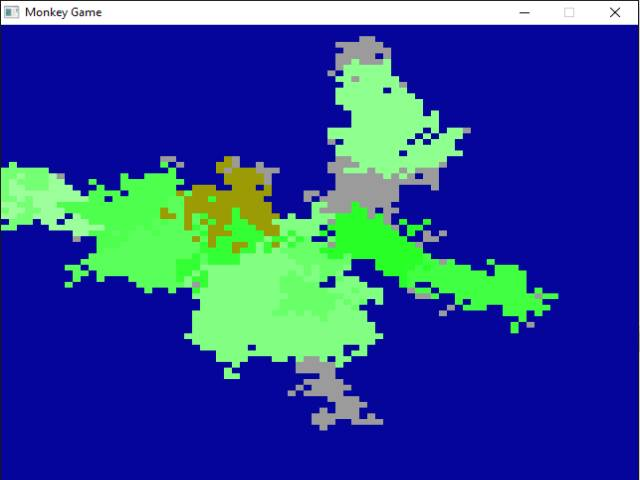-=+=- -=+=- -=+=- -=+=- -=+=- -=+=- -=+=- -=+=- -=+=- -=+=- -=+=- -=+=- -=+=- -=+=- -=+=- -=+=- -=+=- -=+=- -=+=- -=+=- -=+=- -=+=- -=+=- -=+=- -=+=- -=+=- -=+=- -=+=- -=+=- -=+=- (c) WidthPadding Industries 1987 0|568|0 -=+=- -=+=- -=+=- -=+=- -=+=- -=+=- -=+=- -=+=- -=+=- -=+=- -=+=- -=+=- -=+=- -=+=- -=+=- -=+=- -=+=- -=+=- -=+=- -=+=- -=+=- -=+=- -=+=- -=+=- -=+=- -=+=- -=+=- -=+=- -=+=- -=+=-
SoCoder -> Snippet Home -> Generators

PakzCreated : 20 June 2018
Edited : 20 June 2018
Language : Monkey-X

### Map Generator Lines and Zones(grow)

2d Maps

Screenshots## Latest Update

This code(commented) creates maps by creating lines in a stack and drawing these on a map. Each line has a length and a angle. It starts with one line and at random new lines can be added at the current line position each with a new random length and direction.

When the lines have been drawn the generator function grows the lines so they turn into landmasses. Becourse the lines are all connected a single land mass is formed.

Then another zone(grow) set is done. A number of zone numbers are put onto land coordinates and they are also grown creating those biomes.